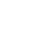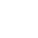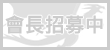LV. 14
GP 94# RE:【討論】巨集程式編寫入門指南

GP5 BP-
※ 引述《matif (Matif)》之銘言
> 不太了解原來發問者的需求
> 但我想應該可以改成如下：
> /run
> local function a(v)
> 　for i = 1, 24 do
> 　　if UnitBuff("player",i)==v then return true end
> 　end
> end
> if not a("XX") then UseSkill(n1,n2) end
> ...

/run
local function a(x, y, z)
for (i = 1, 99) do
if (x == UnitBuff("player", i)) then
return;
end
end
UseSkill(y, z);
end
a("魔法屏障", 3, 5);
a("魔法奧秘", 4, 9);
a("泉水祝福", 3, 6);
a("抗火結界", 2, 6);
...............

/run local function a(x,y,z)for i=1,99 do if x==UnitBuff("player",i)then return end end UseSkill(y,z)end a("魔法屏障",3,5)a("魔法奧秘",4,9)a("泉水祝福",3,6)a("抗火結界",2,6)face基於日前微軟官方表示 Internet Explorer 不再支援新的網路標準，可能無法使用新的應用程式來呈現網站內容，在瀏覽器支援度及網站安全性的雙重考量下，為了讓巴友們有更好的使用體驗，巴哈姆特即將於 2019年9月2日 停止支援 Internet Explorer 瀏覽器的頁面呈現和功能。